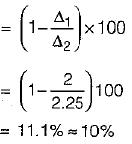Courses

# Test: Tension, Compression & Shear - 3

## 10 Questions MCQ Test Topicwise Question Bank for Mechanical Engineering | Test: Tension, Compression & Shear - 3

Description
This mock test of Test: Tension, Compression & Shear - 3 for Mechanical Engineering helps you for every Mechanical Engineering entrance exam. This contains 10 Multiple Choice Questions for Mechanical Engineering Test: Tension, Compression & Shear - 3 (mcq) to study with solutions a complete question bank. The solved questions answers in this Test: Tension, Compression & Shear - 3 quiz give you a good mix of easy questions and tough questions. Mechanical Engineering students definitely take this Test: Tension, Compression & Shear - 3 exercise for a better result in the exam. You can find other Test: Tension, Compression & Shear - 3 extra questions, long questions & short questions for Mechanical Engineering on EduRev as well by searching above.
QUESTION: 1

### A bar having a cross-sectional area of 700 mm2 is subjected to axial loads at the positions indicated. The value of stress in the segment QR is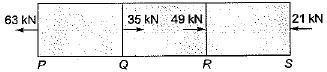Solution:

Free body diagram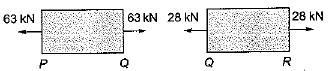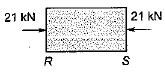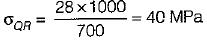QUESTION: 2

### A bar of 20 mm diameter is tested in tension. It is observed that when a load of 30 kN is applied the extension measured over a gauge length of 200 mm is 0.12 mm and contraction in diameter is 0.0036 mm. The Poisson’s ratio is

Solution: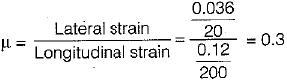QUESTION: 3

### If all the dimensions of a prismatic bar of square cross-section suspended freely from the ceiling of a roof are doubled then the total’elongation produced by its own weight will increase

Solution:

The area of bar will become 4 times, and the volume as well as weight will increase 8 times. So increase in elongation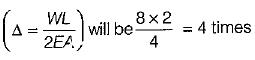QUESTION: 4

A round bar made of same material consists of 3 parts each of 100 mm length having diameters of 40 mm, 50 mm and 60 mm, respectively, if the bar is subjected to an axial load of 10 kN, the total elongation of the bar in mm would be (E is the modulus of elasticity in kN/mm2)

Solution:

Total elongation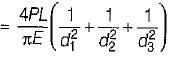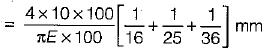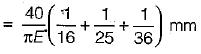QUESTION: 5

Two identical circular rods of same diameter and same length are subjected to same magnitude of axial tensile force. One of the rods is made out of mild steel having the modulus of elasticity of 206 GPa. The other rod is made out of cast iron having the modulus of elasticity of 100 GPa. Assume both the materials to be homogeneous and isotropic and the axial force causes the same amount of uniform stress in both the rods. The stresses developed are with in the proportional limit of the respective materials. Which of the following observations is correct?

Solution:

Given,
EMS = 206 GPa
ECI = 100GPa
We know that,
ΔL = (PL/AE)
Here, P, L and A is same for both the bars
∴ L ∝ 1/E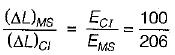= 0.485 < 1

QUESTION: 6

A uniform, slender cylindrical rod is made of a homogeneous and isotropic material. The rod rests on a frictionless surface. The rod is heated uniformly. If the radial and longitudinal thermal stresses are represented by σr and σz respectively, then

Solution:

Since the rod is free to expand hence no thermal stresses will be generated in radial well as longitudinal direction.
∴ σ= 0 and σz = 0

QUESTION: 7

A brass bar of solid section is encased in a steel tube as shown in the diagram below: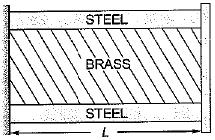The coefficient of expansion of steel is 11.2 x 10-6 per °C and the coefficient of expansion of brass is 16.5 x 10-6 per °C. The composite bar is heated through 60°C. Now consider the following statements:
1. The stress in brass will be tensile.
2. The stress in steel will be tensile.
3. The stress in steel will be compressive.
4. The stress in brass will be compressive.
Q. Which of these statements are correct?

Solution:

Since coefficient of thermal expansion for brass is more than steel hence brass will expand more than steel at the same temperature rise and due to greater expansion of brass, it will try to stretch the steel. Hence tensile stresses will be generated on in steel while compressive stresses will be generated in brass.

QUESTION: 8

A uniform bar lying in the x - direction is subjected to pure bending. Which one of the following tensors represents the strain variation when bending moment is about the z-axis (p, q and r constants)?

Solution:
QUESTION: 9

Assuming E = 160 GPa and G = 100 GPa for a material a strain tensor is given as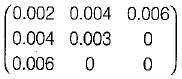The shear stress, τxy is

Solution:

Strain Tensor =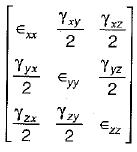On comparison, we get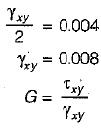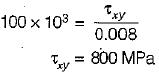QUESTION: 10

A bar of circular cross-section varies uniformly from a cross-section 2D to D. If extension of the bar is calculated treating it as a bar of average diameter, then the percentage error will be

Solution: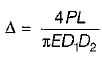Actually,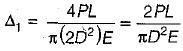The average diameter of bar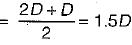Approximate extension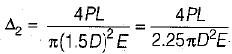% Error